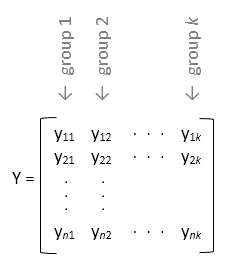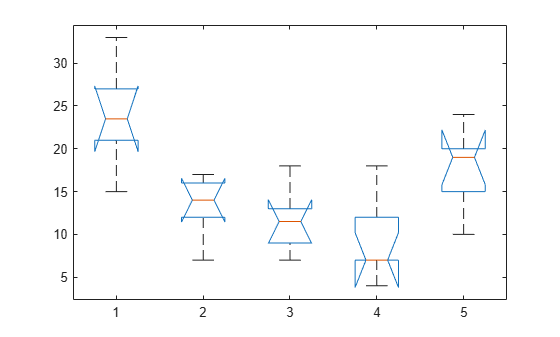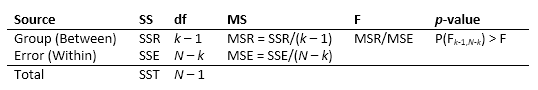## One-Way ANOVA

### Introduction to One-Way ANOVA

You can use the function `anova1` to perform one-way analysis of variance (ANOVA). The purpose of one-way ANOVA is to determine whether data from several groups (levels) of a factor have a common mean. That is, one-way ANOVA enables you to find out whether different groups of an independent variable have different effects on the response variable y. Suppose, a hospital wants to determine if the two new proposed scheduling methods reduce patient wait times more than the old way of scheduling appointments. In this case, the independent variable is the scheduling method, and the response variable is the waiting time of the patients.

One-way ANOVA is a simple special case of the linear model. The one-way ANOVA form of the model is

`${y}_{ij}={\alpha }_{j}+{\epsilon }_{ij}$`

with the following assumptions:

• yij is an observation, in which i represents the observation number, and j represents a different group (level) of the variable y. All yij are independent.

• αj represents the population mean for the jth group (level or treatment).

• εij is the random error, independent and normally distributed, with zero mean and constant variance, i.e., εij ~ N(0,σ2).

This model is also called the means model. The model assumes that the columns of y are the constant αj plus the error component εij. ANOVA helps determine if the constants are all the same.

ANOVA tests the hypothesis that all group means are equal (${H}_{0}:{\alpha }_{1}={\alpha }_{2}=...={\alpha }_{k}$) against the alternative hypothesis that at least one group is different from the others (${H}_{1}:{\alpha }_{i}\ne {\alpha }_{j}$ for at least one i and j). `anova1(y)` tests the equality of column means for the data in matrix `y`, where each column is a different group and has the same number of observations (i.e., a balanced design). `anova1(y,group)` tests the equality of group means, specified in `group`, for the data in vector or matrix `y`. In this case, each group or column can have a different number of observations (i.e., an unbalanced design).

ANOVA is based on the assumption that all sample populations are normally distributed. It is known to be robust to modest violations of this assumption. You can check the normality assumption visually by using a normality plot (`normplot`). Alternatively, you can use one of the Statistics and Machine Learning Toolbox™ functions that checks for normality: the Anderson-Darling test (`adtest`), the chi-squared goodness of fit test (`chi2gof`), the Jarque-Bera test (`jbtest`), or the Lilliefors test (`lillietest`).

### Prepare Data for One-Way ANOVA

You can provide sample data as a vector or a matrix.

• If the sample data is in a vector, `y`, then you must provide grouping information using the `group` input variable: `anova1(y,group)`.

`group` must be a numeric vector, logical vector, categorical vector, character array, string array, or cell array of character vectors, with one name for each element of `y`. The `anova1` function treats the `y` values corresponding to the same value of `group` as part of the same group. For example,Use this design when groups have different numbers of elements (unbalanced ANOVA).

• If the sample data is in a matrix, `y`, providing the group information is optional.

• If you do not specify the input variable `group`, then `anova1` treats each column of `y` as a separate group, and evaluates whether the population means of the columns are equal. For example,Use this form of design when each group has the same number of elements (balanced ANOVA).

• If you specify the input variable `group`, then each element in `group` represents a group name for the corresponding column in `y`. The `anova1` function treats the columns with the same group name as part of the same group. For example,`anova1` ignores any `NaN` values in `y`. Also, if `group` contains empty or `NaN` values, `anova1` ignores the corresponding observations in `y`. The `anova1` function performs balanced ANOVA if each group has the same number of observations after the function disregards empty or `NaN` values. Otherwise, `anova1` performs unbalanced ANOVA.

### Perform One-Way ANOVA

This example shows how to perform one-way ANOVA to determine whether data from several groups have a common mean.

Load and display the sample data.

```load hogg hogg```
```hogg = 6×5 24 14 11 7 19 15 7 9 7 24 21 12 7 4 19 27 17 13 7 15 33 14 12 12 10 23 16 18 18 20 ```

The data comes from a Hogg and Ledolter (1987) study on bacteria counts in shipments of milk. The columns of the matrix `hogg` represent different shipments. The rows are bacteria counts from cartons of milk chosen randomly from each shipment.

Test if some shipments have higher counts than others. By default, `anova1` returns two figures. One is the standard ANOVA table, and the other one is the box plots of data by group.

`[p,tbl,stats] = anova1(hogg);``p`
```p = 1.1971e-04 ```

The small p-value of about 0.0001 indicates that the bacteria counts from the different shipments are not the same.

You can get some graphical assurance that the means are different by looking at the box plots. The notches, however, compare the medians, not the means. For more information on this display, see `boxplot`.

View the standard ANOVA table. `anova1` saves the standard ANOVA table as a cell array in the output argument `tbl`.

`tbl`
```tbl=4×6 cell array {'Source' } {'SS' } {'df'} {'MS' } {'F' } {'Prob>F' } {'Columns'} {[ 803.0000]} {[ 4]} {[200.7500]} {[ 9.0076]} {[1.1971e-04]} {'Error' } {[ 557.1667]} {} {[ 22.2867]} {0x0 double} {0x0 double } {'Total' } {[1.3602e+03]} {} {0x0 double} {0x0 double} {0x0 double } ```

Save the F-statistic value in the variable `Fstat`.

`Fstat = tbl{2,5}`
```Fstat = 9.0076 ```

View the statistics necessary to make a multiple pairwise comparison of group means. `anova1` saves these statistics in the structure `stats`.

`stats`
```stats = struct with fields: gnames: [5x1 char] n: [6 6 6 6 6] source: 'anova1' means: [23.8333 13.3333 11.6667 9.1667 17.8333] df: 25 s: 4.7209 ```

ANOVA rejects the null hypothesis that all group means are equal, so you can use the multiple comparisons to determine which group means are different from others. To conduct multiple comparison tests, use the function `multcompare`, which accepts `stats` as an input argument. In this example, `anova1` rejects the null hypothesis that the mean bacteria counts from all four shipments are equal to each other, i.e., ${H}_{0}:{\mu }_{1}={\mu }_{2}={\mu }_{3}={\mu }_{4}$.

Perform a multiple comparison test to determine which shipments are different than the others in terms of mean bacteria counts.

`results = multcompare(stats);`The figure also illustrates the same result. The blue bar shows the comparison interval for the first group mean, which does not overlap with the comparison intervals for the second, third, and fourth group means, shown in red. The comparison interval for the mean of fifth group, shown in gray, overlaps with the comparison interval for the first group mean. Hence, the group means for the first and fifth groups are not significantly different from each other.

Display the multiple comparison results in a table.

```tbl = array2table(results,"VariableNames", ... ["Group A","Group B","Lower Limit","A-B","Upper Limit","P-value"])```
```tbl=10×6 table Group A Group B Lower Limit A-B Upper Limit P-value _______ _______ ___________ _______ ___________ _________ 1 2 2.4953 10.5 18.505 0.0059332 1 3 4.1619 12.167 20.171 0.0012925 1 4 6.6619 14.667 22.671 0.0001262 1 5 -2.0047 6 14.005 0.21195 2 3 -6.3381 1.6667 9.6714 0.97193 2 4 -3.8381 4.1667 12.171 0.55436 2 5 -12.505 -4.5 3.5047 0.48062 3 4 -5.5047 2.5 10.505 0.88757 3 5 -14.171 -6.1667 1.8381 0.19049 4 5 -16.671 -8.6667 -0.66193 0.029175 ```

The first two columns show which group means are compared with each other. For example, the first row compares the means for groups 1 and 2. The last column shows the p-values for the tests. The p-values 0.0059, 0.0013, and 0.0001 indicate that the mean bacteria counts in the milk from the first shipment is different from the ones from the second, third, and fourth shipments. The p-value of 0.0292 indicates that the mean bacteria counts in the milk from the fourth shipment is different from the ones from the fifth. The procedure fails to reject the hypotheses that the other group means are different from each other.

### Mathematical Details

ANOVA tests for the difference in the group means by partitioning the total variation in the data into two components:

• Variation of group means from the overall mean, i.e., ${\overline{y}}_{.j}-{\overline{y}}_{..}$ (variation between groups), where ${\overline{y}}_{.j}$ is the sample mean of group j, and ${\overline{y}}_{..}$ is the overall sample mean.

• Variation of observations in each group from their group mean estimates, ${y}_{ij}-{\overline{y}}_{.j}$ (variation within group).

In other words, ANOVA partitions the total sum of squares (SST) into sum of squares due to between-groups effect (SSR) and sum of squared errors(SSE).

`$\underset{SST}{\underbrace{\sum _{i}\sum _{j}{\left({y}_{ij}-{\overline{y}}_{..}\right)}^{2}}}=\underset{SSR}{\underbrace{\sum _{j}{n}_{j}{\left({\overline{y}}_{.j}-{\overline{y}}_{..}\right)}^{2}}}+\underset{SSE}{\underbrace{\sum _{i}\sum _{j}{\left({y}_{ij}-{\overline{y}}_{.j}\right)}^{2}}},$`

where nj is the sample size for the jth group, j = 1, 2, ..., k.

Then ANOVA compares the variation between groups to the variation within groups. If the ratio of between-group variation to within-group variation is significantly high, then you can conclude that the group means are significantly different from each other. You can measure this using a test statistic that has an F-distribution with (k – 1, Nk) degrees of freedom:

`$F=\frac{SSR}{k-1}}{SSE}{N-k}}=\frac{MSR}{MSE}~{F}_{k-1,N-k},$`

where MSR is the mean squared treatment, MSE is the mean squared error, k is the number of groups, and N is the total number of observations. If the p-value for the F-statistic is smaller than the significance level, then the test rejects the null hypothesis that all group means are equal and concludes that at least one of the group means is different from the others. The most common significance levels are 0.05 and 0.01.

#### ANOVA Table

The ANOVA table captures the variability in the model by source, the F-statistic for testing the significance of this variability, and the p-value for deciding on the significance of this variability. The p-value returned by `anova1` depends on assumptions about the random disturbances εij in the model equation. For the p-value to be correct, these disturbances need to be independent, normally distributed, and have constant variance. The standard ANOVA table has this form:`anova1` returns the standard ANOVA table as a cell array with six columns.

ColumnDefinition
`Source`Source of the variability.
`SS`Sum of squares due to each source.
`df`Degrees of freedom associated with each source. Suppose N is the total number of observations and k is the number of groups. Then, Nk is the within-groups degrees of freedom (`Error`), k – 1 is the between-groups degrees of freedom (`Columns`), and N – 1 is the total degrees of freedom: N – 1 = (Nk) + (k – 1).
`MS`Mean squares for each source, which is the ratio `SS/df`.
`F`F-statistic, which is the ratio of the mean squares.
`Prob>F`p-value, which is the probability that the F-statistic can take a value larger than the computed test-statistic value. `anova1` derives this probability from the cdf of the F-distribution.

The rows of the ANOVA table show the variability in the data, divided by the source.

Row (Source)Definition
`Groups` or `Columns`Variability due to the differences among the group means (variability between groups)
`Error`Variability due to the differences between the data in each group and the group mean (variability within groups)
`Total`Total variability

 Wu, C. F. J., and M. Hamada. Experiments: Planning, Analysis, and Parameter Design Optimization, 2000.

 Neter, J., M. H. Kutner, C. J. Nachtsheim, and W. Wasserman. 4th ed. Applied Linear Statistical Models. Irwin Press, 1996.